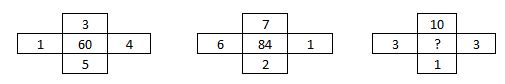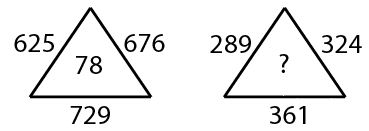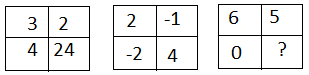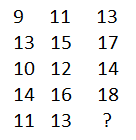0
472

# SSC CGL Series Questions

Download Top-20 Series questions for SSC CGL exam 2020. Most important  Series  questions based on asked questions in previous exam papers for SSC CGL.

Question 1: Which one set of letters when sequentially placed at the gaps in the given letter series shall complete it?
l_b_ub_ubt_blu_tub

a) ubtlu

b) utlub

c) tulbu

d) butlu

Instructions

In the following two questions, which one set of letters when sequentially placed at the gaps in the given letter series shall complete it ?

Question 2: ccbab _ caa _ bccc _ a

a) abb

b) bab

c) bbb

d) baa

Instructions

In the following questions, which one set of letters when sequentially placed at the gaps in the given letter series shall complete it.

Question 3: adb _ ac _ da_cddcb _ dbc _ cbda

a) bccba

b) ccbba

c) cbbaa

Question 4: a_b_ ca_b_c_a_cc

a) acbcab

b) ababca

c) acacab

d) ababac

Instructions

In each of the following questions, which one set of letters/numbers when sequentially placed at the gaps in the given letter series shall complete it?

Question 5: SH _ ELAS _ EELA _ HEELA SHEE _ A

a) HHSS

b) EEHS

c) EHSL

d) ELHA

Question 6: 12 _ 41 _ 34123 __ 234

a) 3241

b) 2134

c) 1432

d) 3212

Question 7: aba _ ba _ ab

a) abbab

b) baabb

c) abbbb

d) bbaba

Instructions

In each of the following questions, select the related word/letters/numbers from the given alternatives.

Question 8: SOCIAL : OCIALS : : DRIVEN : ?

a) VENRID

b) NEVIRD

c) RIVEND

d) VIREND

Instructions

In each of the following questions, a series is given, with one term missing. Choose the correct alternative from the given ones that will complete the series.

Question 9: BMX, DNW, FOV, ? .

a) GHO

b) GPS

c) HPS

d) HPU

Question 10: 655, 637, 622, 610, 601, ?

a) 598

b) 595

c) 596

d) 597

Instructions

In each of the following questions, a series is given with one term missing. Choose the correct alternative from the given ones that will complete the series.

Question 11: TMJ,QNL, NON, KPP, ___?

a) JQR

b) HQR

c) HQQ

d) IQS

Question 12: A, CD, GHI, ?, UVWXYZ

a) LMNP

b) MNOL

c) NOPL

d) MNOP

Instructions

In each of the following questions, select the missing number from the given responses.

Question 13:a) 70

b) 90

c) 100

d) 89

Instructions

In each of the following questions. a series is given, with one term missing. Choose the correct alternative from the given ones that will complete the series.

Question 14: AZBY, CXDW, EVFU, ?

a) SHTG

b) GXHW

c) GTHS

d) STHO

Instructions

In each of the following questions, select the missing number from the given responses.

Question 15:a) 34

b) 54

c) 44

d) 64

Instructions

In the following two questions, a series is given, with one term missing. Choose the correct alternative from the given ones that will complete the series.

Question 16: 3, 5, 9, 17, ?

a) 42

b) 26

c) 65

d) 33

Instructions

In the following five questions, select the missing number from the given responses.

Question 17:a) 1

b) 30

c) 11

d) 0

Question 18:a) 21

b) 22

c) 14

d) 15

Instructions

In each of the following questions, a series is given, with one term missing. Choose the correct alternative from the given ones that will complete the series.

Question 19: 1, 2, 2, 4, 3, 8, 7, 10, ?

a) 11

b) 13

c) 9

d) 8

Question 20: 0, 7, 26, 63, ?

a) 123

b) 87

c) 96

d) 124

The pattern followed is that, in a group of three letters, two alternate words ‘lub’ and ‘tub’ are written.

=> lutub lub tub lutub

=> Ans – (B) : utlub

Expression : ccbab _ caa _ bccc _ a

The pattern followed is that, in a group of 4 letters, the first two letters are repeated.

=> ccba bbca aabc ccba

=> Ans – (B)

Expression : adb _ ac _ da_cddcb _ dbc _ cbda

The pattern is that in a group of 4 letters, there is a combination of first four alphabets, i.e. ‘a,b,c,d’.

=> adbc acbd abcd dcba dbca cbda

=> Ans – (C)

Expression : a_b_ ca_b_c_a_cc

In a group of 5 letters, the term ‘aabcc’ is repeated.

= aabcc  aabcc  aabcc

=> Ans – (C)

Expression : SH _ ELAS _ EELA _ HEELA SHEE _ A

The pattern is that the word ‘SHEELA’ is repeated.

=> SHEELA SHEELA SHEELA SHEELA

=> Ans – (C)

in the given pattern 12 _ 41 _ 34123 _ _ 234

the complete pattern is 1234

hence the blanks will be filled with 3241 from left t right

we are given that SOCIAL : OCIALS : : DRIVEN : ?

in SOCIAL : OCIALS , we can see that first alphabet is shifted to last

and hence the same thing will be done in 2nd case

and hence DRIVEN : RIVEND

here the given series is BMX, DNW, FOV, ?

as we can see that 1st alphabet of every term in series is alternative element starting from B

2nd alphabet if every term series is consecutive alphabets starting from M and 3rd alphabet in every term in the series is retracing back every consecutive alphabet starting from X

and hence the missing pattern is HPU

Let the missing number be x.

The difference between every two consecutive numbers is forming a pattern.

655-637 = 18

637-622 = 15

622-610 = 12

610-601 = 9

As the difference is getting reduced by 3 every time . So 601-x = 6 which implies that the missing number ,x =595

here the given sequence is TMJ,QNL, NON, KPP, ___?

first term of every sequence is retracement of two alphabets starting from T and hence the first term of missing seqience is H

2nd term of every sequence is consecutive alphabets starting from M and hence the 2nd term of missing sequence is Q

3rd term of every sequence is alternative alphabets starting from J and hence last term of missing sequence is R

hence the missing sequence is HQR

here the given series is A, CD, GHI, ?, UVWXYZ

here the pattern is that 1st term is A and then a single alphabet is missed and next two alphabets are written aFter that next two alphabets are missed and next three alphabets are written and so in next case for missing term 3 next terms will be missed i.e. JKL will be missed and next 4 terms will be written i.e MNOP and hence the missing term is MNOP

Here in every pattern the middle term is product of all terms surrounding it

for example , 60 = 1 x 3 x 4 x 5

84 = 6 x 7 x 1 x 2

and hence the missing term in third pattern is = 3 x 10 x 3 x 1 = 90AZBY:

A is the first letter in the alphabetical series. It is followed by the first letter from the last, Z.
B is the second letter in the alphabetical series. It is followed by the second last letter, Y.

The next term starts with ‘C’, the letter following B.

CXDW:

C is the third letter from the start and X is the third letter from the end.
D is the fourth letter from the start and W is the fourth letter from the end.

The next term starts with ‘E’, the letter following D.

EVFU:

E is the fifth letter from the start and V is the fifth letter from the end.
F is the sixth letter from the start and U is the sixth letter from the end.

G is the seventh letter from the start. The seventh letter from the end is T.
H is the eighth letter from the start. The eighth letter from the end is S.
Therefore, the next term must be GTHS. Hence, option C is the right answer.let the missing term be y

here we can see that in first figure

78 = $\surd(625) + \surd(676) + \surd(729)$

and hence same pattern will follow in second figure

y = $\surd(289) + \surd(324) + \surd(361)$ = 54

here the given pattern is 3, 5, 9, 17, ?

let the missing term be y

we can see that

5 – 3 = 2^1

9 -5 = 4 = 2^2

17 – 9 = 8 = 2^3

as we can see that every time the difference between consecutive terms is getting increased by power of 2

and hence , y – 17 = 2^4 = 16

y = 33let the missing term be y

here in 1st figure , the pattern is (3 x 2 x 4) = 24

in 2nd figure , (2 x -1 x -2 ) = 4

in 3rd figure also the same thing will be followed ,

y = (6 x 5 x 0 ) = 0let the missing term be y

here we can see that in 1st column

9 + 4 = 13

13 – 3 = 10

10 + 4 = 14

14 – 3 = 11

now in 2nd column

11 + 4 = 15

15 – 3 = 12

12 + 4 = 16

16 – 3 = 13

hence the same thing will be followed in column 3 and so ,

13 + 4 = 17

17 – 3 = 14

14 + 4 = 18

18 – 3 = 15 and hence y = 15

It is a combination of 2 series, one at odd places and other at even places.

1) : 1,2,3,7, 11,12,13,17     (Difference is 1,1,4,1,1,4,…)

2) : 2,4,8,10, 14,16,20        (Difference is 2,4,2,4,2,….)

=> Ans – (A) : 11

let the missing term be y

here the given series is 0, 7, 26, 63, y

as we can see that :

0 = 0^2 -1

7 = 2^3 – 1

26 = 3^3 – 1

63 = 4^3 – 1

as we can see that all number are equal to cub of consecutive whole numbers minus 1 and hence ,

y = 5^3 – 1= 124